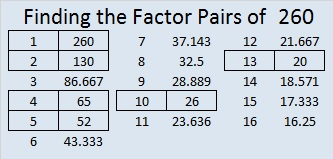# 260 Some Thoughts on Those Four Consecutive Numbers

• 260 is a composite number.
• Prime factorization: 260 = 2 x 2 x 5 x 13, which can be written 260 = (2^2) x 5 x 13
• The exponents in the prime factorization are 2, 1 and 1. Adding one to each and multiplying we get (2 + 1)(1 + 1)(1 + 1) = 3 x 2 x 2 = 12. Therefore 260 has 12 factors.
• Factors of 260: 1, 2, 4, 5, 10, 13, 20, 26, 52, 65, 130, 260
• Factor pairs: 260 = 1 x 260, 2 x 130, 4 x 65, 5 x 52, 10 x 26, or 13 x 20
• Taking the factor pair with the largest square number factor, we get √260 = (√4)(√65) = 2√65 ≈ 16.125Recently I wrote about the smallest four-consecutive-numbers whose square roots could all be simplified. The same numbers were also the smallest four consecutive numbers to have the same number of factors.Each of those numbers had 6 factors, and guess what, ANY number with exactly 6 factors can have its square root simplified. The prime factorization of ANY number with exactly 6 factors can be expressed in one of the three following ways:Since numbers with six factors always have a prime factor raised to a power greater than one, they can always have their square roots simplified. The fact that those four consecutive numbers have the same number of factors makes them extraordinary; that they all can have their square roots simplified is merely the natural consequence of that extraordinary fact.

## 3 thoughts on “260 Some Thoughts on Those Four Consecutive Numbers”

1.Paula

“ANY number with exactly 6 factors can have its square root simplified:” !!! My new fact for the day…er…week…thank you.

2.wbhs62

This continues to bemuse and delight me. While I have no idea what I’m talking about there seems no reason why there there isn’t a chain of five, six, or more consecutive numbers with exactly six divisors. Indeed, dare I suggest that somewhere within the infinite set of integers there is an infinitely long chain of consecutive numbers with exactly six divisors? Gulp.

•ivasallay

I appreciate your enthusiasm! I am very interested in seeing if there could be five, six, or seven consecutive numbers with exactly six divisors, but I already know a string of eight or any number greater than eight is impossible. I’ll write about why I know that soon.

This site uses Akismet to reduce spam. Learn how your comment data is processed.